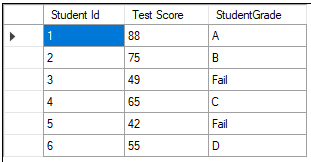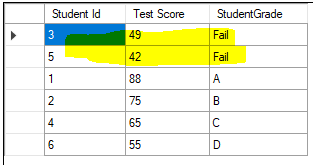# Case Statement

The CASE statement is like an if...then...else condition in other programming languages, it is more closely aligned with Switch statement such as switch statement in c#. Like the IF and SWITCH statement, the CASE statement selects one sequence of statements to execute. The Case statement contains one or more WHEN ... THEN clauses and it is completed with an optional ELSE clause and a required END keyword.

```DROP TABLE IF EXISTS StudentScores; CREATE TABLE StudentScores ( StudentId Integer , TestName Text , Score Real ); INSERT INTO StudentScores values (1,'Math', 88); INSERT INTO StudentScores values (2,'Math', 75); INSERT INTO StudentScores values (3,'Math', 49); INSERT INTO StudentScores values (4,'Math', 65); INSERT INTO StudentScores values (5,'Math', 42); INSERT INTO StudentScores values (6,'Math', 55); ```

The following sql statement present how to use case when with optional else, it adds a column with values based on Score column.

```SELECT StudentId [Student Id], Score [Test Score] , CASE WHEN Score >= 80 THEN 'A' WHEN Score >=70 THEN 'B' WHEN Score >=60 THEN 'C' WHEN Score >=50 THEN 'D' ELSE 'Fail' END as [StudentGrade] FROM StudentScores; ```Conditional Order by using Case When statement, where we want to bring students who have failed on top of the list.

```SELECT StudentId [Student Id], Score [Test Score] , CASE WHEN Score >= 80 THEN 'A' WHEN Score >=70 THEN 'B' WHEN Score >=60 THEN 'C' WHEN Score >=50 THEN 'D' ELSE 'Fail' END as [StudentGrade] FROM StudentScores ORDER BY CASE WHEN [StudentGrade] = 'Fail' THEN 1 ELSE 2 END; ```